Science, Maths & Technology

### Become an OU studentEveryday maths 1 (Wales)

Start this free course now. Just create an account and sign in. Enrol and complete the course for a free statement of participation or digital badge if available.

# 4.1 Instruments of measure

To measure a very small amount, you might use a teaspoon. This is the same as 5 millilitres (ml).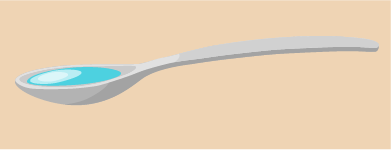Figure 26 A teaspoon

To measure larger amounts, you would probably use a measuring jug of some kind. Measuring jugs are often labelled in millilitres, especially newer ones, and they can come in different sizes: some can measure up to 500 ml of liquid and others up to 1 litre (1 000 ml). Some may hold more or less than this.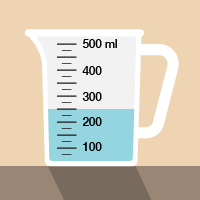Figure 27 A measuring jug

Now take a look at the following example.

## Example: Measuring liquids

If you had to measure out 350 ml of juice for a recipe, where would the liquid come to in this jug?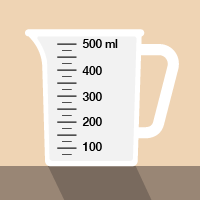Figure 28 Measuring liquids in a measuring jug

### Method

There are three marks on the jug between 300 ml and 400 ml. These mark 325, 350 and 375 ml. So you need to fill the jug to the middle mark (remember to look for the level where the liquid touches the scale). You may have to hold a jug up to eye level to measure the amount as accurately as you can.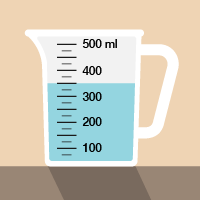Figure 29 Measuring liquids in a measuring jug (answer)

Now try the following activity. Remember to check your answers once you have completed the questions.

## Activity 15: Looking at capacity (volume)

Now that you have seen the example, have a go at the following activity;

1. How much coffee or tea does a cup you usually drink out of hold? Estimate the volume first, and write down your estimate. Next, fill your cup with water and then pour the water into a measuring jug. (Hint: A standard bottle of water holds 500 ml. A can of of pop is 330 ml.)
2. A scientist has to measure 2.8 ml of liquid in this syringe. Where should the liquid come to?Figure 30 A syringe
3. A plumber has drained water from a faulty central heating system into a set of measuring jugs. How many litres in total has the plumber drained from the system? Notice how on these measuring jugs, the scale is marked up in fractions of a litre rather than in millilitres.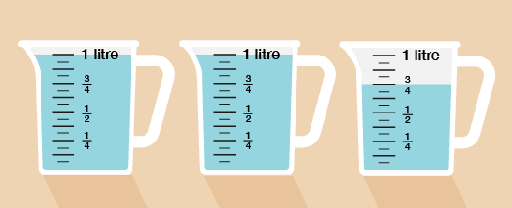Figure 31 Three measuring jugs